|

# 淋浴房界的“黑科技”?一文看懂恒洁卫浴新品

现代人工作生活节奏快，加班熬夜更是常事。一整天辛苦的伏案工作后，没有比回家洗个热水澡更解压的事儿了，淋浴房可谓是不可或缺的利器了，不仅提升卫生间格调，还能减少打扫烦恼，而干湿分离的淋浴环境更是能大大地提升居家幸福感。但不同于马桶等标准化浴室配件，传统的淋浴房屏风并无标准化规格，选购及订制流程既花时间，又容易出错，给忙碌的上班族带来了不少麻烦。

现在，有一款淋浴房屏风产品，自带“黑科技”，轻松解决了这一烦恼。今天，小狐就带各位看官一起走进恒洁易至EASY BOX淋浴房，看看它是如何实力C位出道的！

说到淋浴房，小狐脑海里就浮现了四个词——省心、省力、高颜值、科技感！没想到，恒洁易至EASY BOX淋浴房正好就满足了小狐对淋浴房的所有幻想！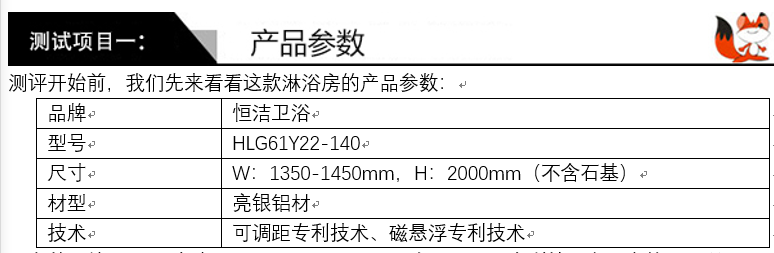测评开始前，我们先来看看这款淋浴房的产品参数

从参数上就可以看到，恒洁易至EASY BOX淋浴房在选材和专利技术方面真的是表现不凡了！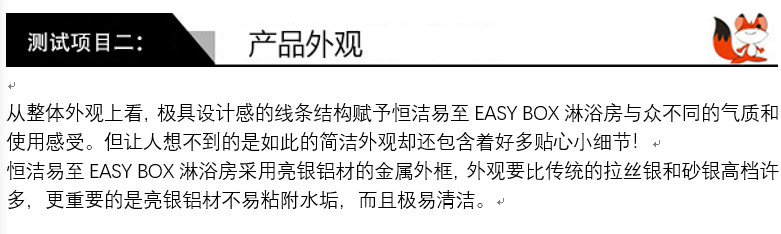从整体外观上看，极具设计感的线条结构赋予恒洁易至EASY BOX淋浴房与众不同的气质和使用感受。但让人想不到的是如此的简洁外观却还包含着好多贴心小细节！

恒洁易至EASY BOX淋浴房采用亮银铝材的金属外框，外观要比传统的拉丝银和砂银高档许多，更重要的是亮银铝材不易粘附水垢，而且极易清洁。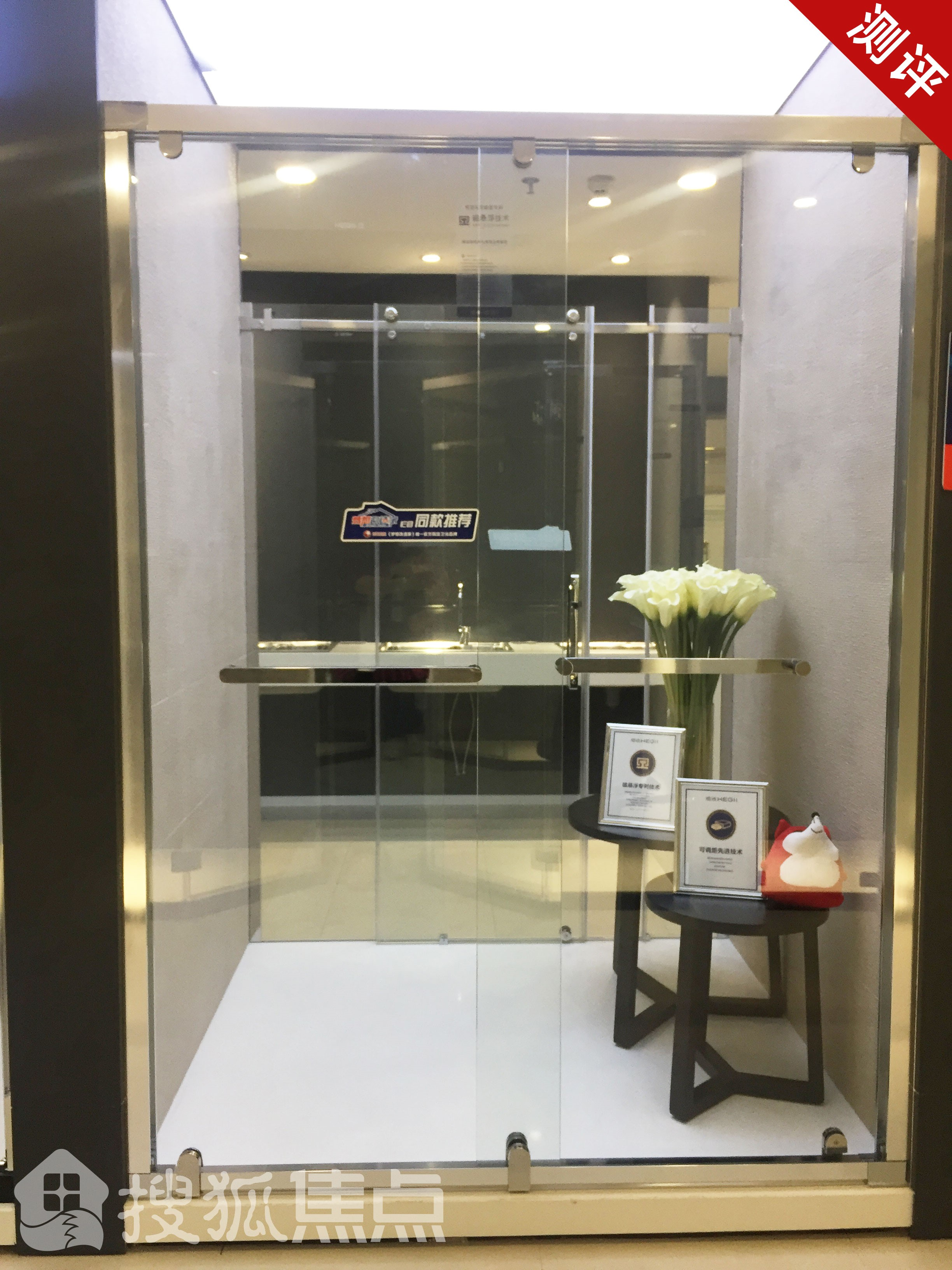恒洁易至EASY BOX淋浴房的石基厚度达50mm，而且还有白色、白金砂、黑色和黑金砂四种颜色可选，轻松让淋浴房与整体家装风格保持一致。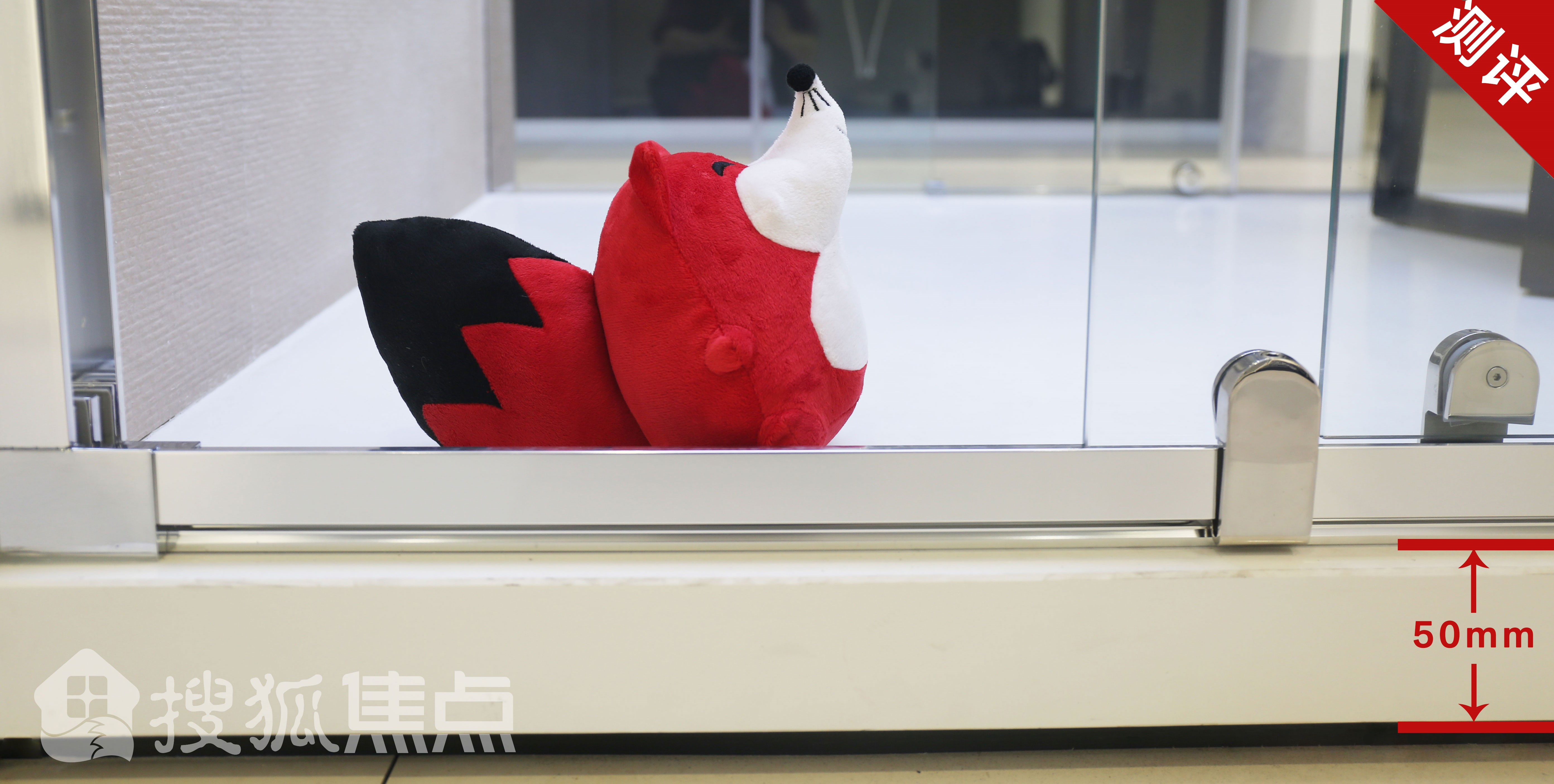作为一款人性化的产品，淋浴房采用双向推拉门的设计，一字型的把手设计简洁大方，线条流畅。双向多功能把手抓手感强，不易滑手。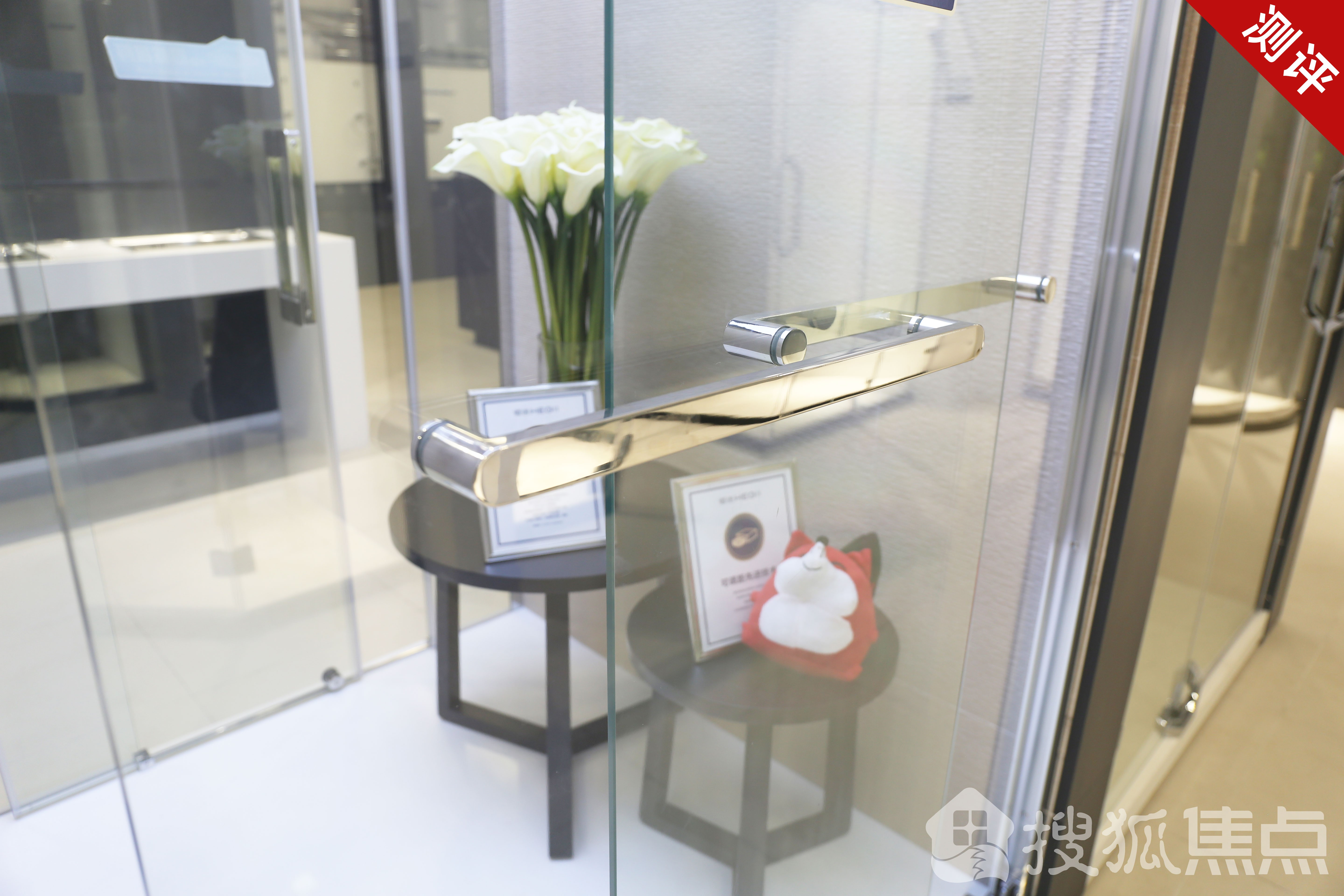另外，不知小伙伴们有没有发现，传统的淋浴房都是要通过胶条达到防水目的，但恒洁易至EASY BOX淋浴房采用了免胶条的设计，最大程度的保留了玻璃的通透感，同时还杜绝了胶条老化发黄的隐患，让恒洁易至EASY BOX淋浴房一直颜值在线！

最后，恒洁易至EASY BOX淋浴房还加入了一体式下防撞块设计，有效缓冲关闭时玻璃挂击铝型的力度，更能保护玻璃，提高耐用性。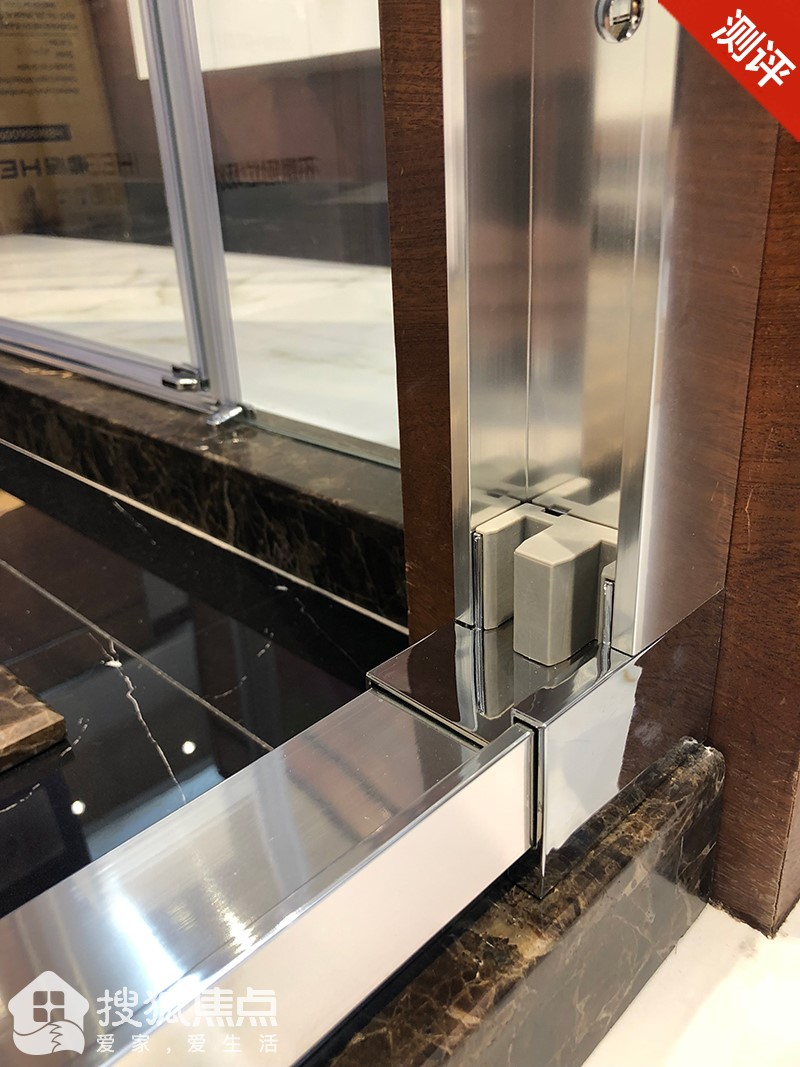恒洁易至EASY BOX淋浴房设计的贴心细节再加上精简的选购、定制流程，让你无论是在安装还是在日常使用过程中都能感到从容和舒适。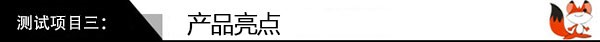现代人生活节奏忙碌，追求的是高效、便捷的体验，作为一款优秀产品，一定是内外兼修的，于细节处体现产品的匠心设计。恒洁易至EASY BOX淋浴房使用的“黑科技”，正是从细节处颠覆原有的淋浴体验，带来极致享受。

可调距专利技术

淋浴间看似设计简单，但购买、安装却是一门大工程，要经历繁琐的测量与沟通，，不仅费时、费力，如果出现误差，还需要返回重制，对于忙碌的现代人来说，真是个令人头疼的问题。恒洁易至EASY BOX淋浴房采用了突破领界的可调距专利技术，在100mm的调距内实现一字型、互推门式淋浴房标准化订制，免去反复测量、误差返修的烦恼。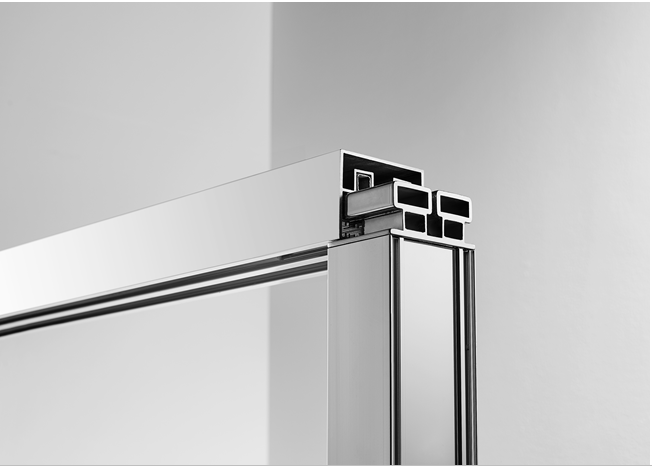2. 磁悬浮专利技术

借鉴磁悬浮列车运行原理的基础上，恒洁易至EASY BOX淋浴房采用了上轨磁悬技术，完美的分解了下轮的承重，从而减少摩擦力，实现推拉平稳、轻盈、静音。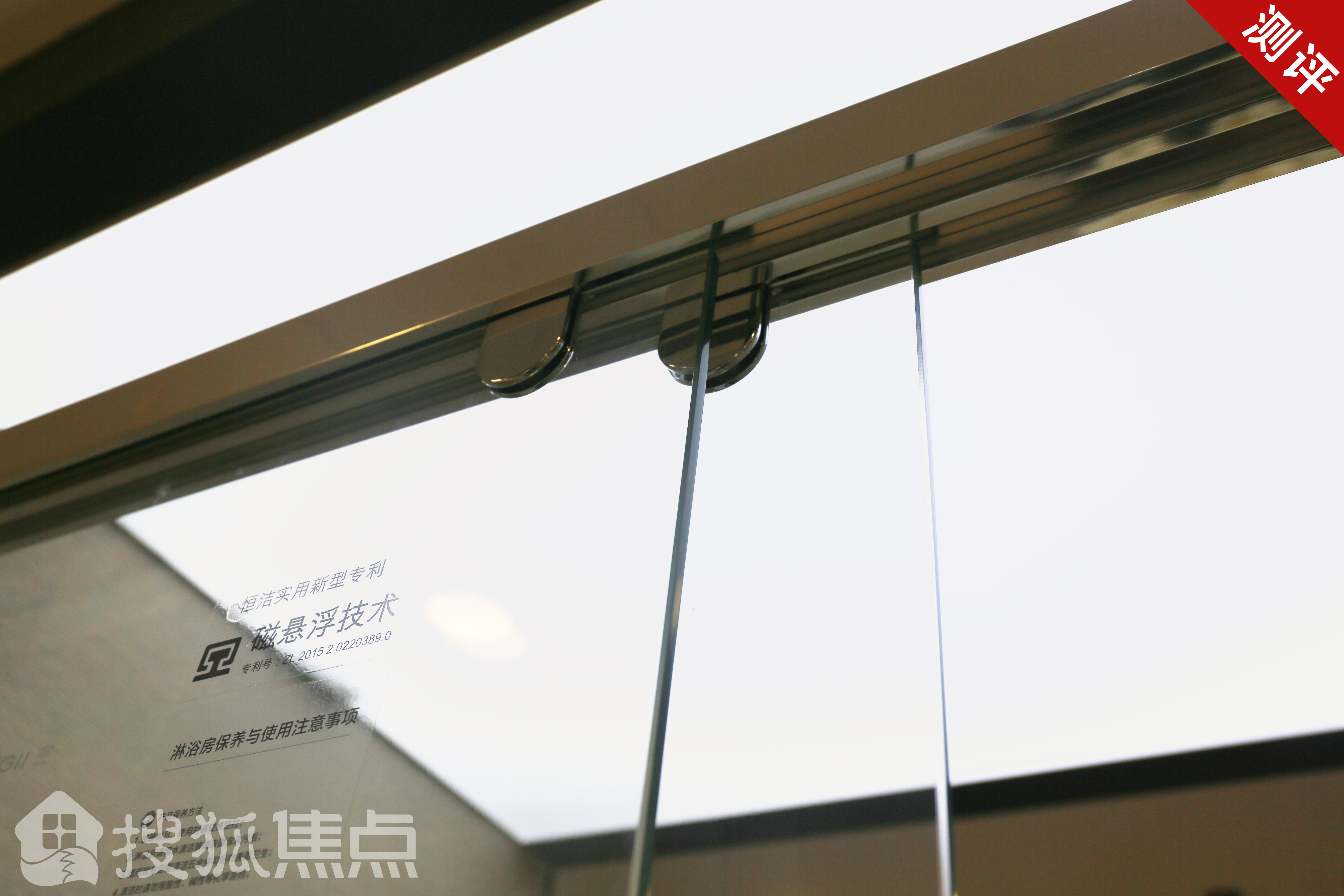3. 防渗水设计

一个完美的淋浴体验，最重要的是实现干湿分离。恒洁易至EASY BOX淋浴房除了使用可调距技术实现边框100%贴合墙面，即便墙面不垂直也能杜绝渗透风险，还通过免打胶的设计，有效地防止了墙面渗水，保持整个淋浴环境的整洁美观。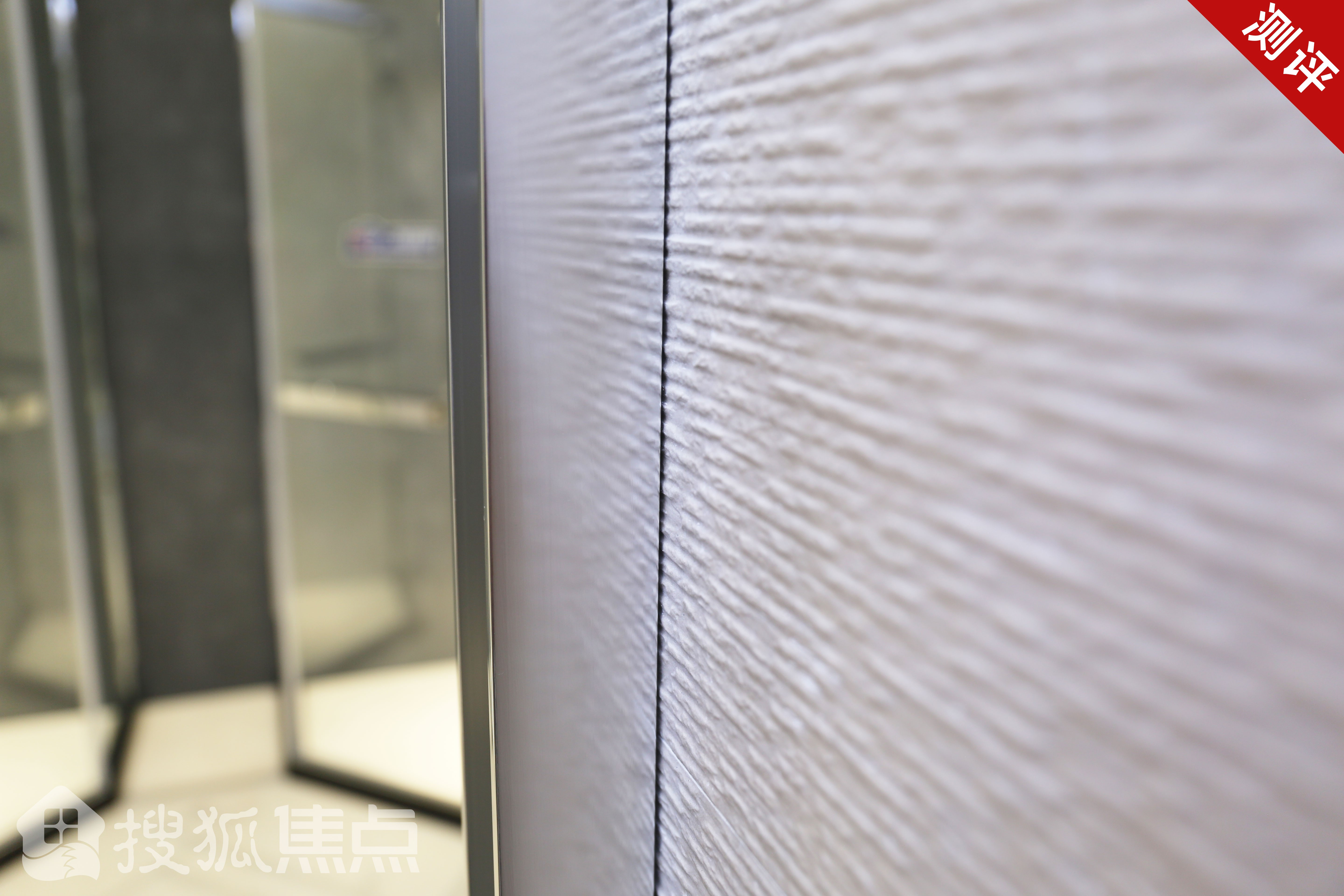4. 高性价比、轻定制化服务

有了可调距技术的“黑科技”加持，恒洁易至EASY BOX淋浴房实现了标准化生产，摆脱了非标定制淋浴房漫长的生产周期以及昂贵的订制费用。工作人员告诉小狐，只需5天，便可实现即买、即提、即安装，真是太方便了！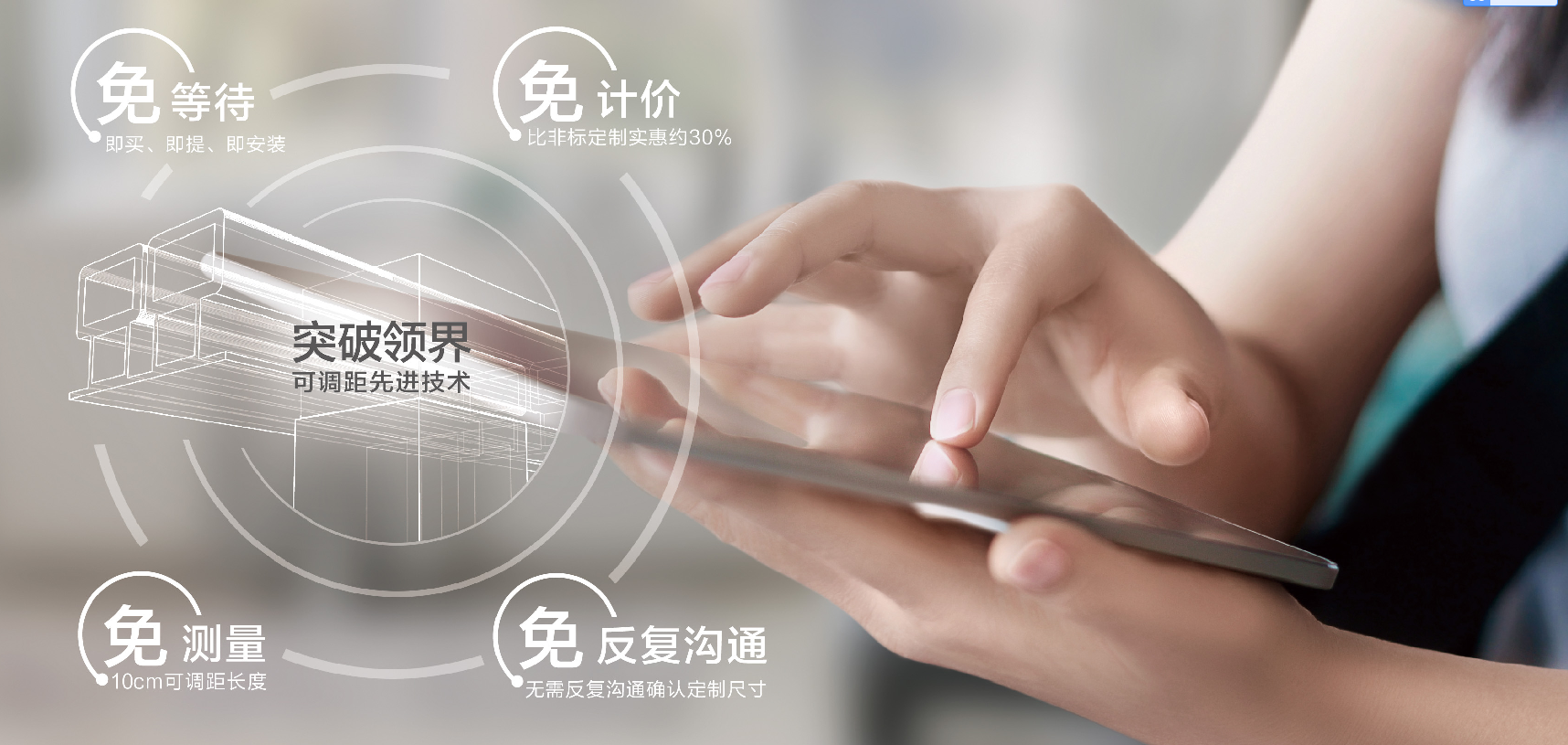恒洁易至EASY BOX淋浴房采用了可调距和磁悬浮两大专利技术，以其酷炫的“黑科技”，为每一个用户轻松带来升级化的便捷舒适淋浴体验。高端大气的简洁设计，更为淋浴体验增添了一重视觉享受。最后，小狐祝各位看官能够在恒洁易至EASY BOX淋浴房里：`声明：本文由入驻焦点开放平台的作者撰写，除焦点官方账号外，观点仅代表作者本人，不代表焦点立场错误信息举报电话： 400-099-0099，邮箱：jubao@vip.sohu.com，或点此进行意见反馈，或点此进行举报投诉。`A B C D E F G H J K L M N P Q R S T W X Y Z
A - B - C - D - E
• A
• 鞍山
• 安庆
• 安阳
• 安顺
• 安康
• 澳门
• B
• 北京
• 保定
• 包头
• 巴彦淖尔
• 本溪
• 蚌埠
• 亳州
• 滨州
• 北海
• 百色
• 巴中
• 毕节
• 保山
• 宝鸡
• 白银
• 巴州
• C
• 承德
• 沧州
• 长治
• 赤峰
• 朝阳
• 长春
• 常州
• 滁州
• 池州
• 长沙
• 常德
• 郴州
• 潮州
• 崇左
• 重庆
• 成都
• 楚雄
• 昌都
• 慈溪
• 常熟
• D
• 大同
• 大连
• 丹东
• 大庆
• 东营
• 德州
• 东莞
• 德阳
• 达州
• 大理
• 德宏
• 定西
• 儋州
• 东平
• E
• 鄂尔多斯
• 鄂州
• 恩施
F - G - H - I - J
• F
• 抚顺
• 阜新
• 阜阳
• 福州
• 抚州
• 佛山
• 防城港
• G
• 赣州
• 广州
• 桂林
• 贵港
• 广元
• 广安
• 贵阳
• 固原
• H
• 邯郸
• 衡水
• 呼和浩特
• 呼伦贝尔
• 葫芦岛
• 哈尔滨
• 黑河
• 淮安
• 杭州
• 湖州
• 合肥
• 淮南
• 淮北
• 黄山
• 菏泽
• 鹤壁
• 黄石
• 黄冈
• 衡阳
• 怀化
• 惠州
• 河源
• 贺州
• 河池
• 海口
• 红河
• 汉中
• 海东
• 怀来
• I
• J
• 晋中
• 锦州
• 吉林
• 鸡西
• 佳木斯
• 嘉兴
• 金华
• 景德镇
• 九江
• 吉安
• 济南
• 济宁
• 焦作
• 荆门
• 荆州
• 江门
• 揭阳
• 金昌
• 酒泉
• 嘉峪关
K - L - M - N - P
• K
• 开封
• 昆明
• 昆山
• L
• 廊坊
• 临汾
• 辽阳
• 连云港
• 丽水
• 六安
• 龙岩
• 莱芜
• 临沂
• 聊城
• 洛阳
• 漯河
• 娄底
• 柳州
• 来宾
• 泸州
• 乐山
• 六盘水
• 丽江
• 临沧
• 拉萨
• 林芝
• 兰州
• 陇南
• M
• 牡丹江
• 马鞍山
• 茂名
• 梅州
• 绵阳
• 眉山
• N
• 南京
• 南通
• 宁波
• 南平
• 宁德
• 南昌
• 南阳
• 南宁
• 内江
• 南充
• P
• 盘锦
• 莆田
• 平顶山
• 濮阳
• 攀枝花
• 普洱
• 平凉
Q - R - S - T - W
• Q
• 秦皇岛
• 齐齐哈尔
• 衢州
• 泉州
• 青岛
• 清远
• 钦州
• 黔南
• 曲靖
• 庆阳
• R
• 日照
• 日喀则
• S
• 石家庄
• 沈阳
• 双鸭山
• 绥化
• 上海
• 苏州
• 宿迁
• 绍兴
• 宿州
• 三明
• 上饶
• 三门峡
• 商丘
• 十堰
• 随州
• 邵阳
• 韶关
• 深圳
• 汕头
• 汕尾
• 三亚
• 三沙
• 遂宁
• 山南
• 商洛
• 石嘴山
• T
• 天津
• 唐山
• 太原
• 通辽
• 铁岭
• 泰州
• 台州
• 铜陵
• 泰安
• 铜仁
• 铜川
• 天水
• 天门
• W
• 乌海
• 乌兰察布
• 无锡
• 温州
• 芜湖
• 潍坊
• 威海
• 武汉
• 梧州
• 渭南
• 武威
• 吴忠
• 乌鲁木齐
X - Y - Z
• X
• 邢台
• 徐州
• 宣城
• 厦门
• 新乡
• 许昌
• 信阳
• 襄阳
• 孝感
• 咸宁
• 湘潭
• 湘西
• 西双版纳
• 西安
• 咸阳
• 西宁
• 仙桃
• 西昌
• Y
• 运城
• 营口
• 盐城
• 扬州
• 鹰潭
• 宜春
• 烟台
• 宜昌
• 岳阳
• 益阳
• 永州
• 阳江
• 云浮
• 玉林
• 宜宾
• 雅安
• 玉溪
• 延安
• 榆林
• 银川
• Z
• 张家口
• 镇江
• 舟山
• 漳州
• 淄博
• 枣庄
• 郑州
• 周口
• 驻马店
• 株洲
• 张家界
• 珠海
• 湛江
• 肇庆
• 中山
• 自贡
• 资阳
• 遵义
• 昭通
• 张掖
• 中卫

1室1厅1厨1卫1阳台

1
2
3
4
5

0
1
2

1

1

0
1
2
3报名成功，资料已提交审核A B C D E F G H J K L M N P Q R S T W X Y Z
A - B - C - D - E
• A
• 鞍山
• 安庆
• 安阳
• 安顺
• 安康
• 澳门
• B
• 北京
• 保定
• 包头
• 巴彦淖尔
• 本溪
• 蚌埠
• 亳州
• 滨州
• 北海
• 百色
• 巴中
• 毕节
• 保山
• 宝鸡
• 白银
• 巴州
• C
• 承德
• 沧州
• 长治
• 赤峰
• 朝阳
• 长春
• 常州
• 滁州
• 池州
• 长沙
• 常德
• 郴州
• 潮州
• 崇左
• 重庆
• 成都
• 楚雄
• 昌都
• 慈溪
• 常熟
• D
• 大同
• 大连
• 丹东
• 大庆
• 东营
• 德州
• 东莞
• 德阳
• 达州
• 大理
• 德宏
• 定西
• 儋州
• 东平
• E
• 鄂尔多斯
• 鄂州
• 恩施
F - G - H - I - J
• F
• 抚顺
• 阜新
• 阜阳
• 福州
• 抚州
• 佛山
• 防城港
• G
• 赣州
• 广州
• 桂林
• 贵港
• 广元
• 广安
• 贵阳
• 固原
• H
• 邯郸
• 衡水
• 呼和浩特
• 呼伦贝尔
• 葫芦岛
• 哈尔滨
• 黑河
• 淮安
• 杭州
• 湖州
• 合肥
• 淮南
• 淮北
• 黄山
• 菏泽
• 鹤壁
• 黄石
• 黄冈
• 衡阳
• 怀化
• 惠州
• 河源
• 贺州
• 河池
• 海口
• 红河
• 汉中
• 海东
• 怀来
• I
• J
• 晋中
• 锦州
• 吉林
• 鸡西
• 佳木斯
• 嘉兴
• 金华
• 景德镇
• 九江
• 吉安
• 济南
• 济宁
• 焦作
• 荆门
• 荆州
• 江门
• 揭阳
• 金昌
• 酒泉
• 嘉峪关
K - L - M - N - P
• K
• 开封
• 昆明
• 昆山
• L
• 廊坊
• 临汾
• 辽阳
• 连云港
• 丽水
• 六安
• 龙岩
• 莱芜
• 临沂
• 聊城
• 洛阳
• 漯河
• 娄底
• 柳州
• 来宾
• 泸州
• 乐山
• 六盘水
• 丽江
• 临沧
• 拉萨
• 林芝
• 兰州
• 陇南
• M
• 牡丹江
• 马鞍山
• 茂名
• 梅州
• 绵阳
• 眉山
• N
• 南京
• 南通
• 宁波
• 南平
• 宁德
• 南昌
• 南阳
• 南宁
• 内江
• 南充
• P
• 盘锦
• 莆田
• 平顶山
• 濮阳
• 攀枝花
• 普洱
• 平凉
Q - R - S - T - W
• Q
• 秦皇岛
• 齐齐哈尔
• 衢州
• 泉州
• 青岛
• 清远
• 钦州
• 黔南
• 曲靖
• 庆阳
• R
• 日照
• 日喀则
• S
• 石家庄
• 沈阳
• 双鸭山
• 绥化
• 上海
• 苏州
• 宿迁
• 绍兴
• 宿州
• 三明
• 上饶
• 三门峡
• 商丘
• 十堰
• 随州
• 邵阳
• 韶关
• 深圳
• 汕头
• 汕尾
• 三亚
• 三沙
• 遂宁
• 山南
• 商洛
• 石嘴山
• T
• 天津
• 唐山
• 太原
• 通辽
• 铁岭
• 泰州
• 台州
• 铜陵
• 泰安
• 铜仁
• 铜川
• 天水
• 天门
• W
• 乌海
• 乌兰察布
• 无锡
• 温州
• 芜湖
• 潍坊
• 威海
• 武汉
• 梧州
• 渭南
• 武威
• 吴忠
• 乌鲁木齐
X - Y - Z
• X
• 邢台
• 徐州
• 宣城
• 厦门
• 新乡
• 许昌
• 信阳
• 襄阳
• 孝感
• 咸宁
• 湘潭
• 湘西
• 西双版纳
• 西安
• 咸阳
• 西宁
• 仙桃
• 西昌
• Y
• 运城
• 营口
• 盐城
• 扬州
• 鹰潭
• 宜春
• 烟台
• 宜昌
• 岳阳
• 益阳
• 永州
• 阳江
• 云浮
• 玉林
• 宜宾
• 雅安
• 玉溪
• 延安
• 榆林
• 银川
• Z
• 张家口
• 镇江
• 舟山
• 漳州
• 淄博
• 枣庄
• 郑州
• 周口
• 驻马店
• 株洲
• 张家界
• 珠海
• 湛江
• 肇庆
• 中山
• 自贡
• 资阳
• 遵义
• 昭通
• 张掖
• 中卫• 手机• 分享
• 设计
免费设计
• 计算器
装修计算器
• 入驻
合作入驻
• 联系
联系我们
• 置顶
返回顶部